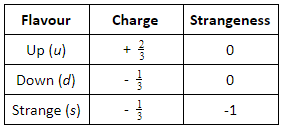# Quarks & Anti-Quarks

## Introduction

In the classification of subatomic particles, as illustrated in Figure 1, Hadrons (Mesons and Baryons) are made up of three smaller particles called quarks. The types of quark are: up (u), down (d) and strange (s). These three types of quarks are also called the three flavours of quark. The combination of these quarks forms Mesons and Baryons. In this article, some important properties of these subatomic particles, and the law governing these properties during particle interaction, are explained.Figure 1: Classification of Particles

A. Up, Down and Strange quarks

The three quark flavours are defined by their charge and strangeness, as shown in the following table. There are three antiquarks: u, d and s. These antiquarks have opposite values of charge and strangeness.B. Charge

Charges of a subatomic particle are defined as fractions of the charge possessed by the elements formed by those particles. The charge of a subatomic particle is in units of e, or the charge of a proton, which is approximatelyCoulombs. So, protons have charge +1, and electrons, -1, using units of e. Protons are composed of two up quarks (u) and one down quark (d), so the total charge is +1. Similarly, neutrons are composed of two down quarks (d) and one up quark (u), so the total charge is 0.

C. Conservation Laws

The conservation law of particle interaction states that, during particle interactions, the following are conserved (the number before the interaction must equal the number after the interaction).

• Charge
• Baryon number
• Lepton number
• Strangeness.

D. Baryon Number

Baryons are characterised by a Baryon number, B of 1. Their antiparticles, called antibaryons, have a Baryon number of −1. An atom containing, for example, one proton and one neutron (each with a Baryon number of 1) has a Baryon number of 2.

E. Lepton Number

A Lepton number is a conserved quantum number representing the difference between the number of Leptons and the number of antileptons in an elementary particle. According to the conservation of law of particle interaction, the total Lepton number before a reaction is equal to the total Lepton number after the reaction.

F. Summary

• Hadrons (Mesons and Baryons) are made up of three smaller particles called quarks.
• The three types of quark are up (u), down (d) and strange (s).
• The charge of a subatomic particle is defined as a fraction of the charge possessed by the elements formed by those particles.
• According to the conservation law of particle interaction, the total Lepton number, Baryon number, charge and strangeness before a reaction is equal to the respective values after the reaction.
• Baryons have a Baryon number of 1. Their antiparticles, called antibaryons, have a Baryon number of −1.
• A Lepton number is a conserved quantum number representing the difference between the number of Leptons and the number of antileptons in an elementary particle.
• Protons are composed of two up quarks (u) and one down quark (d). Neutrons are composed of two down quarks (d) and one up quark (u).